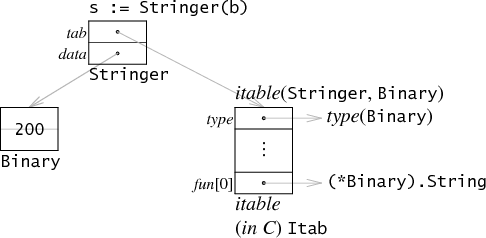Golang 接口與反射知識要點

1. 接口類型變量

type Stringer interface {
String() string
}

type Binary uint64

func (i Binary) String() string {
return strconv.Uitob64(i.Get(), 2)
}

func (i Binary) Get() uint64 {
return uint64(i)
}

b := Binary(200)
var s Stringer = bGo 是靜態類型語言（statically typed）。一個接口類型的不同變量總是有同樣靜態類型，盡管在運行時，接口變量的保存的實際值會改變。下面例子中，無論 r 被賦予的什么實際值，r 的類型總是 io.Reader。

r = os.Stdin
r = new(bytes.Buffer)
// and so on

2. 類型斷言

tty, err := os.OpenFile("/dev/tty", os.O_RDWR, 0)
if err != nil {
return nil, err
}
r = tty

var w io.Writer
w = r.(io.Writer)

3. 鴨子類型

Go 語言雖然是靜態語言，但在接口類型中使用了鴨子類型。不同于其他鴨子類型語言的是，它實現了在編譯時進行靜態檢查，比如變量是否實現接口方法、調用接口方法時參數個數是否相符，同時也不失鴨子類型帶來的靈活和自由。

4. 反射機制

• 什么是反射機制？

• 使用反射的場景？

Go 語言中使用反射的場景：有時候需要根據某些條件決定調用哪個函數，比如根據用戶的輸入來決定，但事先無法不知道接受到的參數是什么類型，全部以 interface{} 類型接受。這時就需要對函數的參數進行反射，在運行期間動態地執行函數。感興趣的讀者可以參考 fmt.Sprint(a ...interface{}) 方法的源碼。

5. reflect 包

TypeOf()、ValueOf()

reflect 包封裝了很多簡單的方法（reflect.TypeOf 和 reflect.ValueOf）來動態獲得類型信息和實際值（reflect.Type，reflect.Value）。

var x float64 = 3.4
fmt.Println("type:", reflect.TypeOf(x))  // 打印 type: float64

fmt.Println("type:", reflect.TypeOf(r))  // 打印 type: *strings.Reader

reflect.TypeOf 方法的函數簽名是 func TypeOf(i interface{}) Type 。它接受任意類型的變量。當我們調用 reflect.TypeOf(x) 時，x 首先存儲在一個空接口類型中，作為傳參。reflect.TypeOf 解析空接口，恢復 x 的類型信息。而調用 reflect.ValueOf 則可以恢復 x 實際值。

var x float64 = 3.4
fmt.Println("value:", reflect.ValueOf(x).String()) // 打印 value: <float64 Value>

Type()、Kind()

reflect.Type 和 reflect.Value 都提供了很多方法支持來操作他們。1. reflect.Value 的 Type() 方法返回實際類型信息；2. reflect.Type 和 reflect.Value 都有 Kind() 方法，來獲得實際值的底層類型，結果對應的是 reflect 包中定義的常量；3. reflect.Value 的那些以類型名為方法名的方法，比如 Int()、Float()，能獲得實際值。

var x float64 = 3.4
v := reflect.ValueOf(x)
fmt.Println("type:", v.Type())
fmt.Println("kind is float64:", v.Kind() == reflect.Float64)
fmt.Println("value:", v.Float())

shell script type: float64 kind is float64: true value: 3.4

func main() {
type MyInt int
var x MyInt = 7
v := reflect.ValueOf(x)
fmt.Println("type:", v.Type())
fmt.Println("kind is int:", v.Kind() == reflect.Int)
fmt.Println("value:", v.Int())
}

shell script type: main.MyInt kind is int: true value: 7

v 調用 Kind() 仍是 reflect.Int，即使 x 的靜態類型是 MyInt 而不是 int。總而言之，Kind() 方法無法區分來自 MyInt 的整數型和 int 型，但 Type() 方法可以

Interface()

Interface() 方法能從 reflect.Value 變量中恢復接口值，是 ValueOf() 的逆向。注意的是，Interface() 方法返回總是靜態類型 interface{}。

6. 反射對象的可設置性

SetXXX(), CanSet()

var x float64 = 3.4
v := reflect.ValueOf(x)
v.SetFloat(7.1)  // will panic: reflect.Value.SetFloat using unaddressable value

var x float64 = 3.4
v := reflect.ValueOf(x)
fmt.Println("settability of v:", v.CanSet())  // settability of v: false

Elem()

var x float64 = 3.4
p := reflect.ValueOf(&x) // Note: take the address of x.
fmt.Println("type of p:", p.Type())  // type of p: *float64
fmt.Println("settability of p:", p.CanSet())  // settability of p: false

// Elem returns the value that the interface v contains
// or that the pointer v points to.
// It panics if v's Kind is not Interface or Ptr.
// It returns the zero Value if v is nil.
func (v Value) Elem() Value
v := p.Elem()
fmt.Println("settability of v:", v.CanSet())  // settability of v: true

v.SetFloat(7.1)
fmt.Println(v.Interface())  // 7.1
fmt.Println(x)  // 7.1

7. Struct 的反射

NumField(), Type.Field(i int)

type T struct {
A int
B string
}

t := T{23, "skidoo"}
s := reflect.ValueOf(&t).Elem()
typeOfT := s.Type()

for i := 0; i < s.NumField(); i++ {
f := s.Field(i)
fmt.Printf("%d: %s %s = %v\n", i,
typeOfT.Field(i).Name, f.Type(), f.Interface())
}

Type.Field(i int) 方法返回字段信息，一個 StructField 類型的對象，包含字段名等。

0: A int = 23
1: B string = skidoo

Value.Field(i int)

T 的字段必須是首字母大寫的才可以設置，因為只有暴露的 struct 字段，才具有可設置性

s.Field(0).SetInt(77)
s.Field(1).SetString("Sunset Strip")
fmt.Println("t is now", t) // t is now {77 Sunset Strip}

Value.Field(i int) 返回 struct s 的字段實際值，所以可以用來設置操作。注意 Type.Field(i int) 和 Value.Field(i int) 的用途區別：前者總是負責和實際類型信息獲取相關的操作，后者是與實際值相關的操作

參考文檔

The Laws of Reflection

Go Data Structures: Interfaces

Go 語言的數據結構：Interfaces

posted @ 2019-10-04 03:48 黃擠擠 閱讀(...) 評論(...) 編輯 收藏